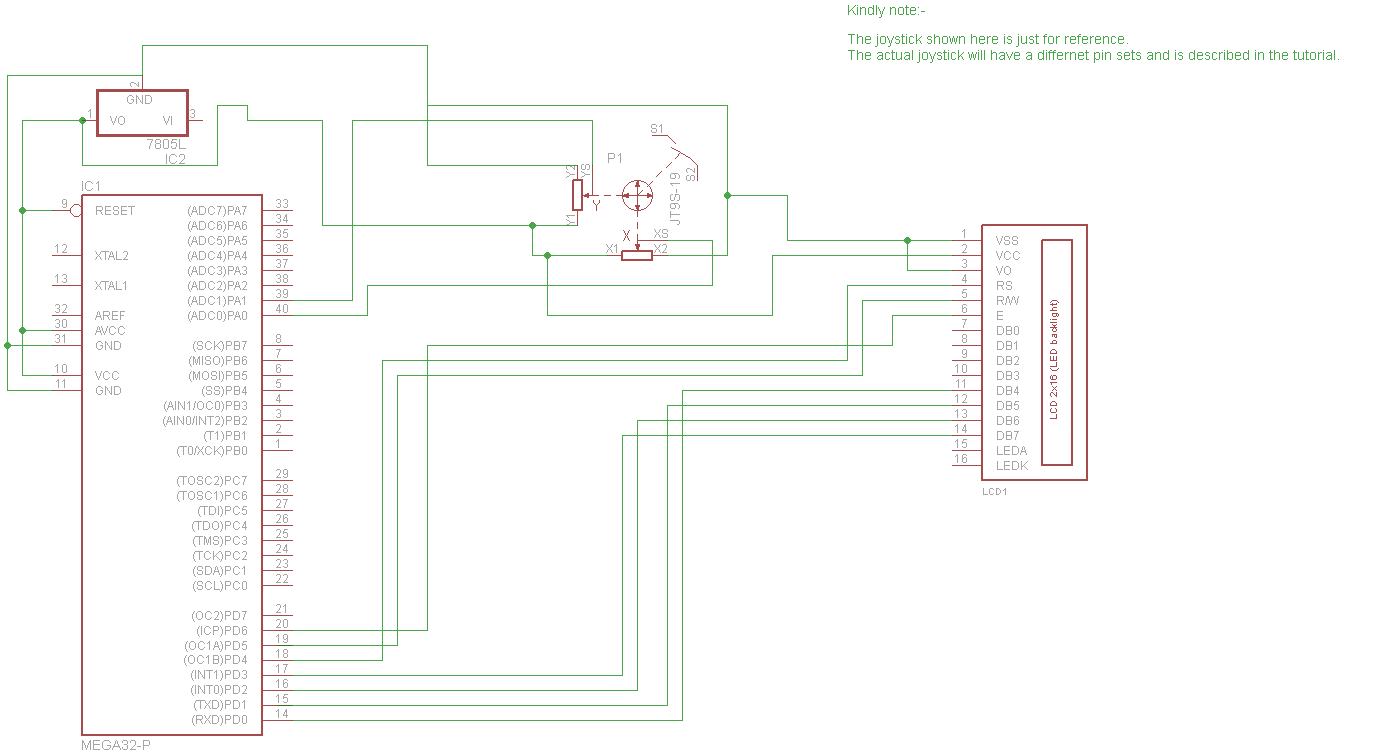# Interfacing analog Joystick with AVR

In this tutorial, we are going to learn something interesting which is already sued by many people to play games. Yes, the analog joystick which is used to control the motion of a vehicle or a character. They are also used to control the movement of an RC car or maybe an RC helicopter. Usually, all the joysticks are two axis joysticks. The ‘y’ axis is used to move the object forward or backward while the ‘x’ axis is generally used to move the object left or right. These joysticks are available both in digital and analog outputs. The digital joystick gives pulse width modulated output but is more costly than the analog output joystick.In this tutorial, we will get the input from the joystick from both the ‘x’ and the ‘y’ axis, convert the analog data into a digital value and then display the data into LCD.  Before starting the tutorial, I will recommend you to read the LCD tutorial, if you haven’t, which can be found in the AVR tutorial section. Also, before displaying the data, there will be some minor calculations involved.

Before moving on to the coding part, let us have a look at the working of a two-axis joystick. The two-axis analog joystick has two variable resistors for two axes. Each variable resistor comprises of three pins, the extreme pins are for VCC and ground, while the middle pin gives the analog output corresponding to the position of the variable resistor on the axis.

Connect both the respective 5v of both axes to 5v input and ground to 0v. Make sure the microcontroller and the joystick both have common ground.  Connect the x-axis output pin to ADC channel 1 (PA0) while the y-axis to ADC channel 2 (PA1). If you are unable to determine which is the x-axis output while which the y-axis output is, connect them randomly. Move the joystick to the extreme position, to check whether you have connected correctly or not. The x-axis should show some value while the y-axis will show value close to zero.

Since we are using the ADC in 10-bit mode, the max value will be 1026 while the minimum value will be 0. For the analog joystick after getting the ADC value, we will subtract the value from 512 to get the value with direction. IF the x-axis value is negative, then the axis is moved to the left, while if the y-axis value is negative, it’s is moved backward. However, the negative sign will still depend on the design of the joystick.

Kindly note the microcontroller is running on a 16MHZ external crystal and the ADC prescaler have been set accordingly. You might have to change the ADC prescaler if you wish to use any other frequency crystal

Example code:

```#ifndef F_CPU
#define F_CPU 16000000UL
#endif
#include <avr/io.h>
#include "lcd.h"        //include LCD Library
#include <util/delay.h>
{
}
{
ch&=0b00000111;         //ANDing to limit input to 7
}
int main(void)
{
char a, b, c;
uint16_t x,y,z;
lcd_init(LCD_DISP_ON);  //INITIALIZE LCD
lcd_clrscr();
while(1)
{
lcd_home();
x=x-512;
y=y-512;
itoa(x,a,10);
itoa(y,b,10);
lcd_puts("x=");     //DISPLAY THE RESULTS ON LCD
lcd_gotoxy(2,0);
lcd_puts(a);
lcd_gotoxy(7,0);
lcd_puts("y=");
lcd_gotoxy(9,0);
lcd_puts(b);
}
}```

Circuit diagram used: